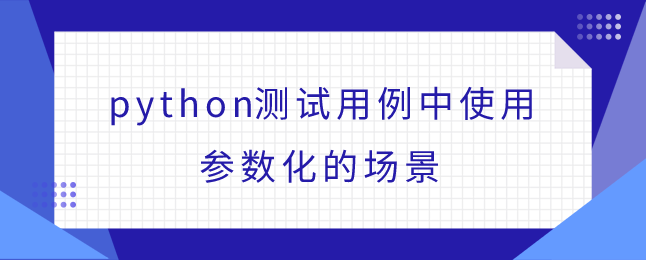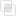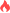python测试用例中使用参数化的场景

python参数化2021-09-22 09:42:40427浏览 · 0收藏 · 0评论1、多个测试用例代码相同，只是测试数据不同，预期结果不同。

2、可以把多个测试用例通过参数化技术合并为一个测试用例。

import unittest
import random

def setUpModule():
print("setUpModule自动调用了")

def tearDownModule():
print("tearDownModule自动调用了")

def my_sum(a, b):
return a + b

class my_test1(unittest.TestCase):
def test_001(self):
num1 = my_sum(5, 6)  # 定义变量num1得到my_sum函数的返回值
self.assertEqual(num1, 11)  # num1里存放的是实际结果,11是预期结果
# 实际结果与预期结果相符,代表测试用例测试通过
# 不相符代表测试用例测试失败

def test_002(self):
num1 = my_sum(0, 3)
self.assertEqual(num1, 3)

def test_003(self):
num1 = my_sum(-3, 7)
self.assertEqual(num1, 4)

def test_004(self):
num1 = my_sum(-4, -20)
self.assertEqual(num1, -24)

# 以上的测试用例,基本是一样的,测试用例的数据和预期结果不同python爬虫urlsplit()方法如何使用python爬虫urljoin()生成链接python爬虫urlencode()方法是什么python爬虫quote()方法有什么用python web框架能够实现的功能python爬虫中aiohttp是什么python字符串怎样劈分python有哪些is开头的字符串方法python去除字符串的前导或后续python Paramiko的SSH用法python TestCase测试用例怎么用python TestSuite测试套件的使用python TestRunner执行测试用例python Fixture的方法级操作python Fixture类级的介绍js事件委托如何理解8306

js中hasOwnProperty的属性用法7452